# The Numbers of Wolfram|Alpha

October 19, 2012 —Comments Off

Wolfram|Alpha answers millions of queries every day. For instance:

These queries represent diverse fields of human activities, spanning mathematics, physics, engineering, chemistry, biology, geography, and some just-geek-fun questions. And despite their diversity, they all have something in common; they contain numbers: integers and reals. Some of the numbers are pure numbers in mathematics, some are counts, and many are quantifying the size, mass, age, et cetera of an object. Some numbers are pretty small, some are pretty large, and some are just one-digit integers.

If one looks at many of the numbers that occur in the queries answered by Wolfram|Alpha, what kind of distribution and what regularities would these numbers have? One regularity is the distribution of the first digit of all numbers, the so-called Benford’s law; an earlier blog post discussed these.

While in principle numbers of any scale exist, in daily life people only use a certain fraction of numbers in size, often between 10-10 and 1010 (from the diameter of an atom measured in meters to, say, the profit of Apple in 2011 measured in US dollars). Occasionally one needs larger numbers, especially for scientific calculations. The SI prefixes cover a range from 10-24 and 1024, spanning 48 orders of magnitude (yotta/yocto). Let’s have a look at the sizes of numbers that users actually used in Wolfram|Alpha queries. We start by looking at integers. As the sample set, I will use a list of the 2.5 billion integers from the recent queries that Wolfram|Alpha did answer.

“God made the integers; all else is the work of man” (according to Kronecker), so let’s see how man uses the integers. We intuitively expect there to be more integers as real numbers in user queries, as they more frequently occur in daily life situations, especially in the form of counting (e.g. 12 eggs, 120 words, 2,000 people). Here is a plot of how many times a number between 0 and 120 occurred. We use a logarithmic plot, as the first few integers are substantially more common than even two-digit integers. One-digits integers (1, 2, 3, 4, …), multiples of 10, and especially the number 100 stand out in their popularity (note that in this logarithmic vertical scale, even a small height difference represents a substantial difference in frequency of occurrence).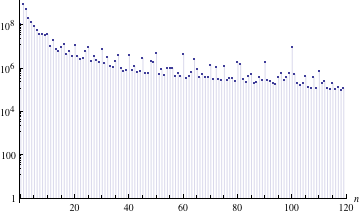And here are the occurrences of the first million integers, this time shown in a log-log plot.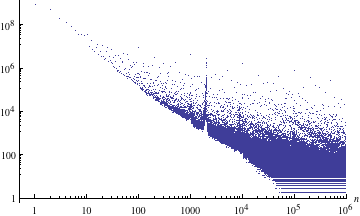The last image shows at least three characteristic features:

1. A power-law-like-decrease in the probability p to find an integer n: p(n)~(nα) where in average α ≈ -1.6. (Some care has to be taken to extract the exponent from noisy data that are conjectured to obey a power law; see Clauset et al, Virkar/Clauset, and Corral et al for details.) The appearance of a Zipf law is not unexpected, as it often appears in many settings where data span many orders of magnitude. (The strictly decreasing lower envelope of the occurrences has a slope of α = -1.75).
2. A quite fractal distribution of the occurrences. Especially for larger numbers, neighboring integers often have a substantially different number of occurrences (sometimes up to six orders of magnitude).
3. The peak-like structure near n ≈ 2000

The characteristic peak around the integer 2,000 is caused by the use of integers as dates. Compared with the use of, say, 2012 in a mathematical calculation, its use in a date is much more common. Zooming in shows this clearly. Recent years (2010 to 2012) are, not unexpectedly, most common (the red line indicates the number 2012). (For the curious: the peaks near 2050 arise from the queries 22014, Moore’s law 2050, and 30th Tuesday in 2051.)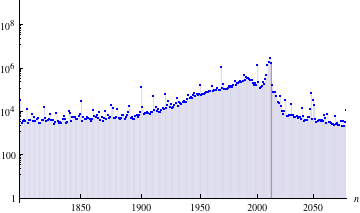The next graphic colors the primes in red. With increasing integer size, the relative probability of being prime increases.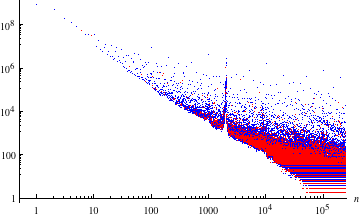Asymptotically, about 38% of all integers typed into Wolfram|Alpha are prime. And more integers are odd than even.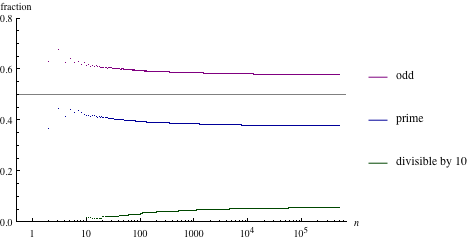While the frequency of an integer in general decreases with size, around powers of ten we have local maxima. The next graphics show the counts for the integers near 100, 1,000, 10,000, and 100,000.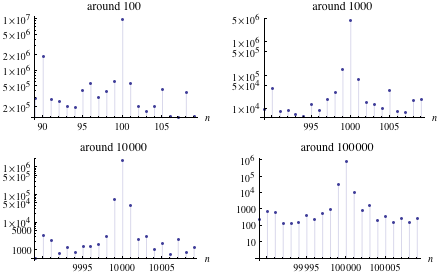Also, powers of two are much more common than neighboring integers.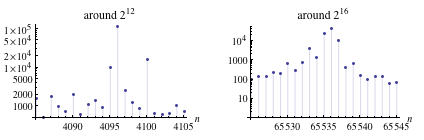Plotting the frequency of q = n mod 10 of all integers shows that multiples of 10 are in general much more common that other integers (we skip all integers less than or equal to 10,000 for this graphic), followed by multiples of 5.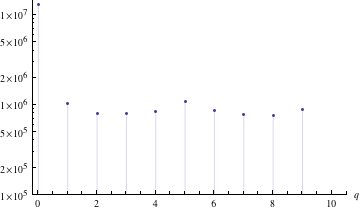Here is a list of the integers that stand out most compared to their left and right neighbors.

We see powers of ten, powers of two, multiples of a dozen, special years (e.g. year 2009), special angles (60 degrees, 360 degrees), the meaning of life, the number of days in a year, Hal 9000, Marty McFly’s 88 mph, 0 degrees Celsius in Kelvins, and others.

10 | 12 | 100 | 20 | 16 | 30 | 25 | 1000 | 50 | 60 | 8 | 40 | 200 | 36 | 45 | 18 | 64 | 32 | 500 | 300 |
10000 | 90 | 120 | 27 | 180 | 400 | 2011 | 125 | 150 | 2000 | 70 | 250 | 80 | 75 | 1968 | 72 |
5000 | 48 | 600 | 365 | 140 | 360 | 110 | 3000 | 128 | 42 | 800 | 144 | 20000 | 160 | 81 | 256 |
900 | 50000 | 88 | 375 | 1200 | 24 | 4000 | 1500 | 240 | 2500 | 154 | 52 | 225 | 1024 | 56 | 700 |
3600 | 2009 | 6000 | 130 | 105 | 350 | 450 | 108 | 135 | 54 | 1988 | 216 | 8000 | 175 | 625 |
1010 | 512 | 96 | 1600 | 220 | 320 | 40000 | 85 | 25000 | 10100 | 170 | 750 | 15000 | 9000 | 1100 | 270 | 273

While many integers occur in a list of 2.5 billion integers, not every integer can occur. So, a naturally occurring question is, “What is the first integer that nobody used?” For the sample set of 2.5 billion integers used, this number turns out to be 69,926 (which is not a valid US ZIP Code and also not a mathematically interesting number, either). Here are the first ten integers that did not occur within the sample (not unexpectedly, none of these are valid US ZIP Codes; US users asked about population and location of about every valid US ZIP Code).

69926 | 70246 | 70635 | 70908 | 70982 | 71501 | 72781 | 72942 | 73519 | 75909

Here is a graphic showing how many integers less than or equal to n did not occur.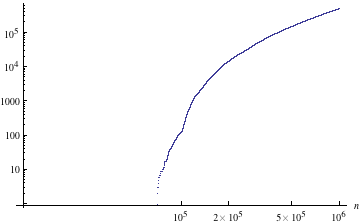Over the much larger interval [1, 10120], the probability of an integer occurring drops quickly with the size of the integer, but less quickly than the above power law for numbers smaller than a million suggested. And the relation between the frequency of an integer and the integer itself is no longer a power law.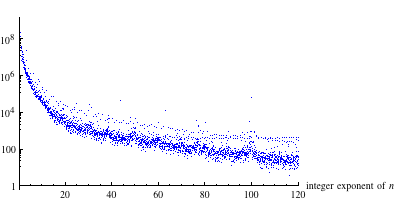Over this large interval [1, 10100], the probability of the occurrence of an integer n scales approximately as p(n) ~ ln(n)α with α ≈ -2.8. (The gray line indicates the power law.)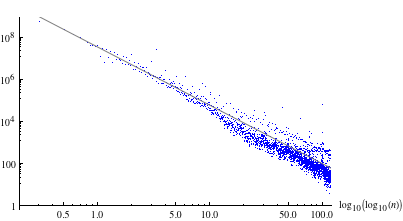The last graphic shows the number of occurrences of the integers. A complementary view is the cumulative one: if one takes all integers n up to a certain size N into account, how many integers has one not taken into account? The following graphic shows this curve.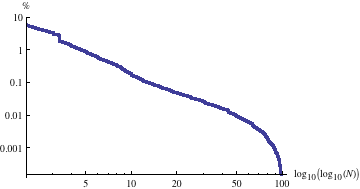Not all integers that occur in Wolfram|Alpha queries are given in fully written out form; often they are written in the form be with base b and exponent e being an integer. The next graphic shows the relative distribution of such powers. One  sees the Zero Barrier (0e) in the East, the Decadrian Wall (10e) in the left half divides the Times Table Valley from the Exponentially Decaying (in popularity) Power Plane, the Cubic Wall (b3) is in the South, and the Diagonal Towers (bb) are along the middle.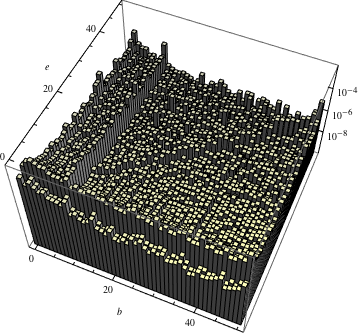In a similar spirit, one could more generally ask which integers n1 and n2 occur frequently together with each other in a query. We display again the frequency of co-occurrence, and we observe that many small integers like to pair with 10 or 100.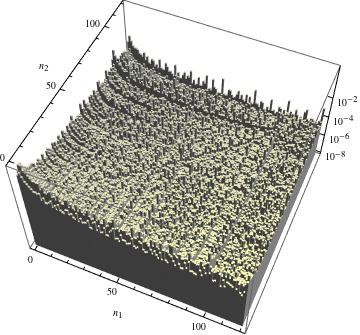Now let us look at some real numbers. Typically there are fewer real numbers than integers occurring in queries, but they come in a larger variety. Using the same query set that contained the above-analyzed 2.5 billion integers yields about 180 million real numbers. The absolute value of real numbers can be greater or less than 1. Here is a distribution of the real numbers by their binned exponent. The pronounced spikes are the real numbers x = 0.5, x = 0.1, and in general at multiples of 0.1.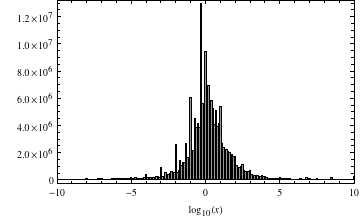The most common real numbers that do not have simple equivalent fractions are 9.81 (from the acceleration due to gravity), 3.14… (from pi), 6.67…10-11 (from the Newtonian gravitational constant), 1.602…10-19 (from the electron charge), 6.626…10-34 (from the Planck constant), 1.38…10-23 (from the Boltzmann constant), and 8.854…10-12 (from the permittivity of free space).

0.5 | 0.1 | 0.2 | 1.5 | 0.9 | 0.01 | 0.25 | 0.3 | 0.4 | 0.05 | 2.5 | 0.8 | 0.6 | 9.8 | 0.7 | 1.2 | 0.75 | 1.1 |
4.5 | 9.81 | 0.02 | 1. | 0.15 | 3.5 | 0.001 | 1.4 | 0.04 | 3.14 | 1.3 | 0.03 | 1.6 | 1.8 | 0.06 | 0.08 |
1.25 | 2.2 | 2.1 | 4.9 | 2. | 2.4 | 7.5 | 0.12 | 1.6 x 10-19 | 0.005 | 0.95 | 6.5 | 8.314 | 5.5 | 1.7 | 0.35 |
0.007 | 0.002 | 1.02 | 2.8 | 3.7 | 0.09 | 0.0001 | 4.2 | 0.025 | 3.2 | 0.45 | 0.5 | 2.3 | 1.9 | 3.6 |
0.125 | 4.8 | 0.99 | 2.7 | 3. | 0.16 | 0.85 | 3.141 | 1.5 | 12.5 | 2.6 | 3.3 | 0.18 | 0.65 | 6.67 x 10-11 |
8.5 | 1.602… x 10-19 | 2.25 | 1.38 x 10-23 | 6.626… x 10-34 | 6. | 1.75 | 1.01 | 3.1 | 5. | 3.4 |
0.55 | 0.14 | 0.11 | 5.8 | 8.854… x 10-12 | 1000. | 0.015 | 2.9

Here is a plot of the distribution over a larger range of reals, ranging over the interval [10-100, 10100], now with a logarithmic vertical scale.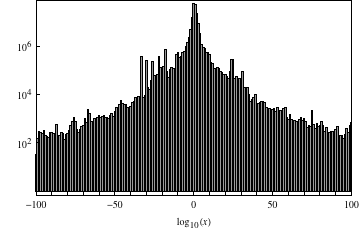And similar to the integers, over the larger interval, the probability of the occurrence of a real number x scales approximately as p(x) ~ |ln(x)|α with α ≈ -2.8. The first graphic shows numbers greater than 1 and the second graphic less than 1 (the gray line indicates again the power law in the exponent).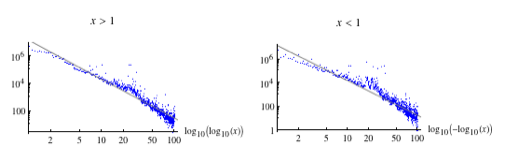The real numbers with just one digit followed by (implied or explicit) zeros (e.g. 0.2, or 0.5) are in general much more common than real numbers with two nonzero digits (e.g. 0.36 or 0.89). The real numbers with just two digits followed by (implied or explicit) zeros (e.g. 0.58 or 0.89) are in general much more common than real numbers with three non-zero digits (e.g. 0.582 or 0.880). And so on with four, five, et cetera nonzero digits. There are some exceptions from this rule, most notably real numbers starting with the digit sequences 314… (leading digits of π) and 667 (three-digit rounded result for 2/3 and the above mentioned Newtonian gravitational constant), and the physical constants mentioned above.

The following graphic shows the frequency of occurrence for four groups of real numbers: in red, the real numbers that have just one nonzero digit; in blue, the real numbers with just two nonzero digits; in green, the real numbers with just three nonzero digits; and in yellow, the real numbers with at least four leading digits. The outliers in green are the digits of π, the first few digits of the acceleration due to gravity on Earth, and multiples of one-eighths. Download the image as a CDF and mouse over the points to see the leading digits. As one would intuitively expect, for every digit added, the number of found real numbers decreases by an order of magnitude. The three thin purple lines are the probabilities for two, three, and four consecutive integers according to the generalized Benford law.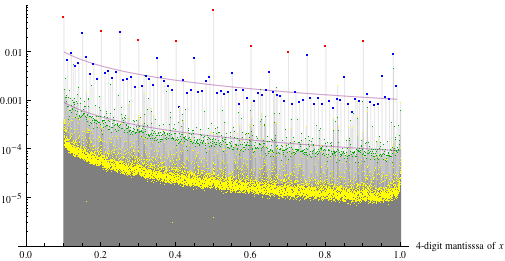The last plot that uses the first four digits of all real numbers shows a lot of details. If we only take the first two digits into account, we have 100 bins, and one clearly sees the integer multiples of 0.1 as well as some of the physical constants emerge.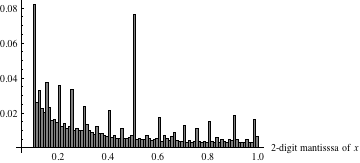This ends our little study of the size distribution of integers and real numbers in Wolfram|Alpha queries. We leave the study of Heap’s law (see Sano et al), meaning how fast new numbers come in, for another time. A study of numbers occurring in web pages was carried out by Dorogovtsev et al. a few years ago; various aspects of the distribution of integers in web pages agree with the above-found distributions in Wolfram|Alpha queries. The importance of special and round numbers was studied by Coupland, Sigurd, and Jansen and Pollmann.

Amazing analysis.

Michael, people used to invest hours in finding Goolge Whacks a few years ago, it might be fun to return an Alpha Whack notification when somebody makes a unique (and otherwise “unimportant”) search!

Posted by Martin Hadley October 19, 2012 at 12:10 pm

Well-written, in-depth, interesting post. This blog is consistently great.

Posted by Allie October 19, 2012 at 12:34 pm

According to the 4-digit mantissa, it seems that the number “0.8314” was used a lot. My idea is that it has something to do with the ideal gas constant, but I wouldn’t imagine it to be used that frequently.

Posted by Matt Smith October 19, 2012 at 12:38 pm

Very nice post Michael. A very similar analysis but using the Online Encyclopaedia of Integer Sequences was done some time ago, with also very similar results (that most anomalies are due to social factors, but that some curves can be modelled by Kolmogorov complexity as a measure of “interestingness”).

Sloane’s Gap. Mathematical and Social Factors Explain the Distribution of Numbers in the OEIS
http://arxiv.org/pdf/1101.4470.pdf
said to appear in the journal of Humanistic Mathematics.

Posted by HZ October 19, 2012 at 6:13 pm

Amazing analysis.

Posted by Bolocan Cristian October 22, 2012 at 11:54 am

Great stuff Michael, very good job!

Posted by Alin November 9, 2012 at 5:18 am

Michael,

My son posed this question to me:

Can all multiples of 2, greater than 2, be written as the sum of 2 primes?

Posted by Bruce Byall December 4, 2012 at 1:22 am

According to the 4-digit mantissa, it seems that the number “0.8314? was used a lot. My idea is that it has something to do with the ideal gas constant, but I wouldn’t imagine it to be used that frequently.

Posted by Daniel May 20, 2013 at 5:34 am

This is an in depth analysis. Great job Michael.

Posted by Francois Magnac October 22, 2013 at 9:40 am

Michael, you have done a terrific job here, I wonder how much time went into this project.. must have been quite a lot.

Posted by Anja Stett December 11, 2013 at 4:08 am

I thought I had waaaaay too much time on my hands. Not comparatively, apparently.

Posted by Me April 24, 2014 at 10:46 pm